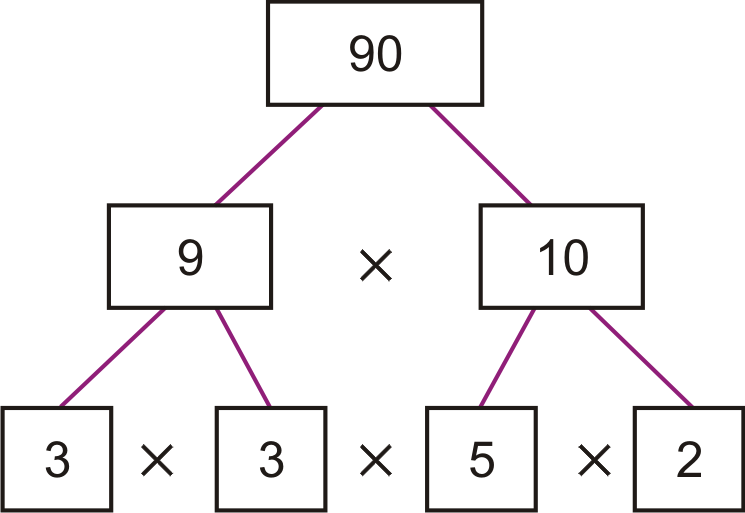Answering the question “what is prime factorization?” first requires defining a few terms.

Factors

Factors are two or more numbers that are multiplied together to get a third number. For example: in the equation “2 x 3 = 6,” the numbers “2” and “3” are both factors.

Prime Numbers

Any number that can only be divided evenly by either itself or by the number 1 is a prime number. Note that only whole numbers that are larger than 1 can be considered prime.

Prime Factorization

Taking into account the above definitions, just about any student should now be able to answer the question “what is a prime factor?” for him- or herself. Prime factorization is simply the act of determining which prime numbers can be multiplied together to make the given number, and prime factors is the term used to define these numbers.

Start Out Small

Let’s look at an example. A question on a student’s math test asks for prime factors of the number 12. One way to get started figuring out the answer is to start from the smallest prime number and work up from there. Not sure how to figure out if a number is prime? Try dividing it by any integers between 1 and the number itself. If any of them yield whole numbers as a result, the number is not prime. Anyone looking for a short-cut can also make use of a prime number table, although that’s whole lot less fun!To get back to the example, let’s start with the lowest prime number: 2. 12 ÷ 2 = 6. The number 6, however, is also not a prime number, so the question hasn’t quite been solved. A new equation must be written out, again starting with the lowest prime number: 6 ÷ 2 = 3. Unlike the answer to the first question, 3 is a prime number. We know this because the only prime number between 3 and 1 is 2, and 3 ÷ 2 = 1.5; not a whole number. So, the answer to the original question would be prime factors of 12 = 2 x 2 x 3. This could also be written out accurately using exponents: 12 = 22 x 3.

Find Any Factors

A second way to solve for prime factors is to break down the original number into any factors that are easier to work with, then break those factors down into prime numbers. Let’s use a slightly harder example this time. Say the student’s math test asked for prime factors of 90 instead of 12. Math novices and enthusiasts alike would find that 90 most easily breaks down into 9 x 10. This leaves us with two much easier to work with numbers. 9 can be divided evenly by 3 (9 = 3 x 3), which we already know from the previous example is a prime number. 10 can be divided by 2 and 5 (10 = 2 x 5), and a little further investigation shows that both of these are also prime numbers. So, the prime factors of 90 = 3 x 3 x 2 x 5.

Factor Trees

Using the above method is easiest when it is visually represented as a factor tree.Keep in mind that each number has its own unique combination of prime factors, so there is only one correct set of prime factors for any given number.